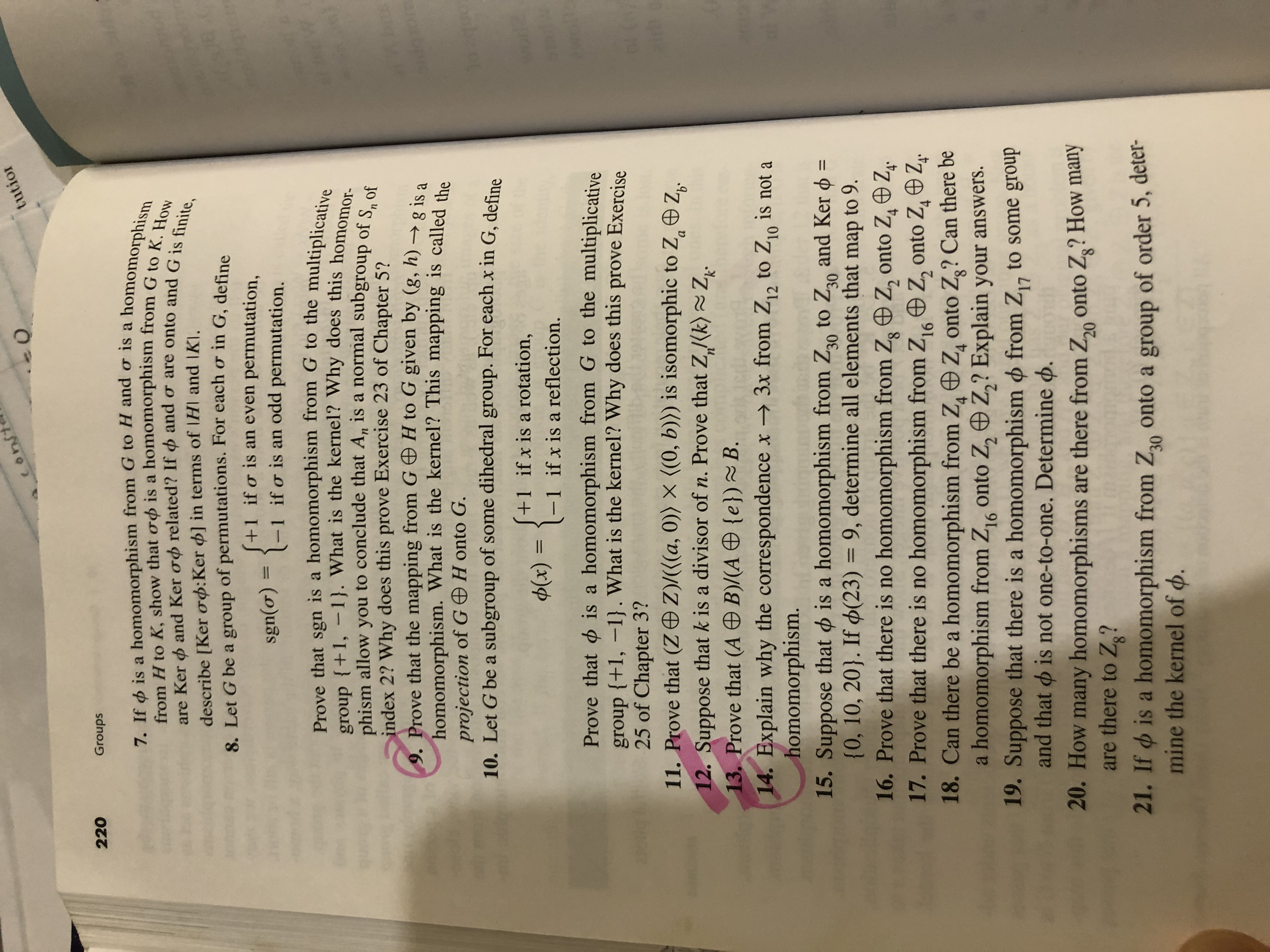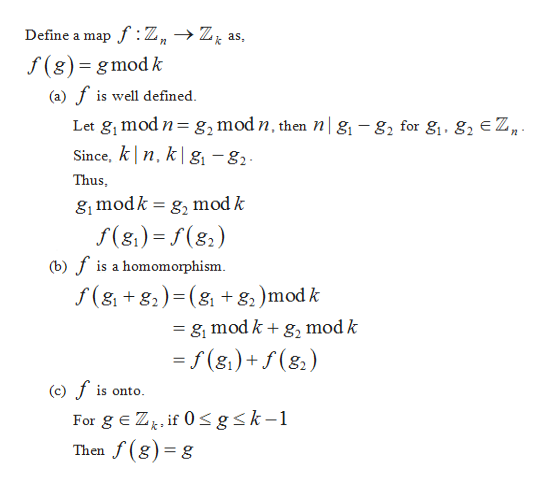# tutiorconst০220Groups7. If is a homomorphism from G to H and o is a homomorphismfrom H to K, show that od is a homomorphism from G to K. Howand Ker od related? If and o are onto and G is finite,are Kerdescribe [Ker od:Ker ] in terms of IHI and IKI.8. Let G be a group of permutations. For each or in G, define+1 if o is an even permutation,if o is an odd permutation.sgn(o)Prove that sgn is a homomorphism from G to the multiplicativegroup +1, -1}. What is the kernel? Why does this homomor-phism allow youindex 2? Why does this prove Exercise 23 of Chapter 5?9. Prove that the mapping from G H to G given by (g, h)-g is ahomomorphism. What is the kernel? This mapping is called theto conclude that A, is a normal subgroup of S ofnprojection of G H onto G.10. Let G be a subgroup of some dihedral group. For each x in G, define202guo+1 if x is a rotation,-1 if x is a reflection.Ф(х)a homomorphism from G to the multiplicativeProve that isgroup {+1, -1}. What is the kernel? Why does this prove Exercise25 of Chapter 3?11. Prove that (Z Z)/(((a, 0)) X ((0, b)))12. Suppose that k is a divisor of n. Prove that Z/(k) Z13. Prove that (A B)/(A {e}) ~ B.14. Explain why the correspondence x3x from Z2 to Z0 is not ahomomorphism.15. Suppose that is a homomorphism from Z0 to Za0 and Ker(0, 10, 20). If d(23) = 9, determine all elements that map to 9.16. Prove that there is no homomorphism from Zg Z, onto Z4 Z17. Prove that there is no homomorphism from Z,16 Z, onto ZgZ18. Can there be a homomorphism from Z, Z onto Z? Can there bea homomorphism from Z16 onto Z, Z,? Explain your answers.19. Suppose that there is a homomorphism from Z1,and that is not one-to-one. Determine .20. How many homomorphisms are there from Z onto Z? How manyare there to Z?21. If is a homomorphism from Z onto a group of order 5, deter-mine the kernel of d.isomorphic to Z®Z.is101130to some group20www

Question
5 views

12help_outlineImage Transcriptionclosetutior const ০ 220 Groups 7. If is a homomorphism from G to H and o is a homomorphism from H to K, show that od is a homomorphism from G to K. How and Ker od related? If and o are onto and G is finite, are Ker describe [Ker od:Ker ] in terms of IHI and IKI. 8. Let G be a group of permutations. For each or in G, define +1 if o is an even permutation, if o is an odd permutation. sgn(o) Prove that sgn is a homomorphism from G to the multiplicative group +1, -1}. What is the kernel? Why does this homomor- phism allow you index 2? Why does this prove Exercise 23 of Chapter 5? 9. Prove that the mapping from G H to G given by (g, h)-g is a homomorphism. What is the kernel? This mapping is called the to conclude that A, is a normal subgroup of S of n projection of G H onto G. 10. Let G be a subgroup of some dihedral group. For each x in G, define 202guo +1 if x is a rotation, -1 if x is a reflection. Ф(х) a homomorphism from G to the multiplicative Prove that is group {+1, -1}. What is the kernel? Why does this prove Exercise 25 of Chapter 3? 11. Prove that (Z Z)/(((a, 0)) X ((0, b))) 12. Suppose that k is a divisor of n. Prove that Z/(k) Z 13. Prove that (A B)/(A {e}) ~ B. 14. Explain why the correspondence x3x from Z2 to Z0 is not a homomorphism. 15. Suppose that is a homomorphism from Z0 to Za0 and Ker (0, 10, 20). If d(23) = 9, determine all elements that map to 9. 16. Prove that there is no homomorphism from Zg Z, onto Z4 Z 17. Prove that there is no homomorphism from Z,16 Z, onto ZgZ 18. Can there be a homomorphism from Z, Z onto Z? Can there be a homomorphism from Z16 onto Z, Z,? Explain your answers. 19. Suppose that there is a homomorphism from Z1, and that is not one-to-one. Determine . 20. How many homomorphisms are there from Z onto Z? How many are there to Z? 21. If is a homomorphism from Z onto a group of order 5, deter- mine the kernel of d. isomorphic to Z®Z. is 10 11 30 to some group 20 www fullscreen
check_circle

Step 1

We can show this as shown below:help_outlineImage TranscriptioncloseDefine a mapf :Z,>Z; as f(g) gmodk (a) fis well defined Let g mod n g2 mod n, then n| g, -g2 for g1. g2 E Z, Since, k n, k|g -82 Thus 8 modk g, mod k f(8)=(8) (b)fis a homomorphism f(gg)(g +82)mod k = g mod k +g, mod k =f(s)+(8) (c)fis onto For gE Zk, if 0 sgsk-1 Then f(g) g fullscreen
Step 2

Evaluate kernel of the...

### Want to see the full answer?

See Solution

#### Want to see this answer and more?

Solutions are written by subject experts who are available 24/7. Questions are typically answered within 1 hour.*

See Solution
*Response times may vary by subject and question.
Tagged in

### Math